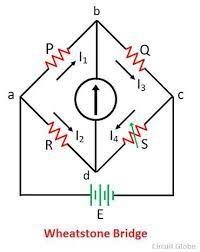Meter bridgeMeter bridge, also called a slide wire bridge, is based on the principle of balanced wheat stone bridge and it is used to find the resistance of an unknown conductor or to compare two unknown resistance. The practical diagram is shown in the below figureMeter Bridge                                                             Balanced condition of                                                                                                                                   wheatstone bridgeThe meter bridge operates under Wheatstone’s principle. Here, four resistors P, Q, R, and S are connected to form the network ABCD. The terminals A and C are connected to a battery, and the terminals B and D are connected to a galvanometer through keys K1 respectively.                                                            In the balancing condition, there is no deflection on the galvanometer. Then, we can apply the balanced form of wheatstone bridge which is as given below :

P/Q = R/S.....................(1)

but in actual instrument ;

P = resistance of length AB

Q = resistance of length BC

R = resistance offered in resistance box

S = unknown resistance

applying in equation 1 , we get

resistance of length AB/ resistance of length BC = R/X

Let l be the length of wire between A and B and then (100- l) is the length between B and C

since we know,

Resistance = ρl / A

As the wire has uniform cross section and ρ is constant, its resistance is proportional to its length i.e.

P α l

Q α  (100- l)

S= (100-l)R/l

The value of S is calculated for different values of R and the mean value of unknown resistance is calculated.